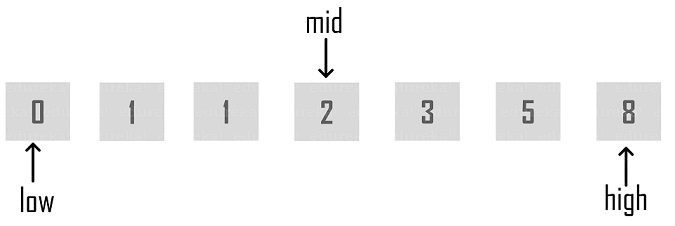# What is Binary Search in Java? How to Implement it?

Last updated on Jun 17,2021 20.2K ViewsA tech enthusiast in Java, Image Processing, Cloud Computing, Hadoop.
12 / 29 Blog from Java Programs

Searching and Sorting algorithms are the popular algorithms in any programming languages. They are the basis to understand the fundamentals of the programming. One such popular searching algorithm is Binary Search in Java. In this article, I will tell you all about its implementation.

Let’s get started!

## What is Binary Search?

Binary Search in Java is a search algorithm that finds the position of a target value within a sorted array. Binary search compares the target value to the middle element of the array. It works only on a sorted set of elements. To use binary search on a collection, the collection must first be sorted.When the binary search is used to perform operations on a sorted set, the number of iterations can always be reduced on the basis of the value that is being searched. You can see in the above snapshot of finding the mid element. The analogy of binary search is to use the information that the array is sorted and reduce the time complexity to O(log n).

## Implementing Binary Search Algorithm

Let’s take a look at the below pseudo code to understand it in a better way.

```Procedure binary_search
A ← sorted array
n ← size of array
x ← value to be searched

Set low = 1
Set high = n

if high < low
EXIT: x does not exist.

set mid = low + ( high - low ) / 2

if A[mid] < x set low = mid + 1 if A[mid]> x
set high = mid - 1

if A[mid] = x
EXIT: x found at location mid
end while

end procedure```

Explanation:

Step 1: First, compare x with the middle element.

Step 2: If x matches with the middle element, then you have to return the mid index.

Step 3: Else, If x is greater than the mid element, then x can only lie in the right side half array after the mid element. Hence you recur the right half.

Step 4: Else, if (x is smaller) then recur for the left half.

That’s how you need to search for the element in the given array.

Let’s now see how to implement a binary search algorithm recursively. Below program demonstrates the same.

## Recursive Binary Search

```public class BinarySearch {
// Java implementation of recursive Binary Search
// Returns index of x if it is present in arr[l..h], else return -1
int binarySearch(int a[], int l, int h, int x)
{
if (h >= l) {
int mid = l + (h - l) / 2;
// If the element is present at the middle itself
if (a[mid] == x)
return mid;
// If element is smaller than mid, then it can only be present in left subarray
if (a[mid] >x)
return binarySearch(arr, l, mid - 1, x);
// Else the element can only be present in right subarray
return binarySearch(arr, mid + 1, h, x);
}
// We reach here when element is not present in array
return -1;
}
public static void main(String args[])
{
BinarySearch ob = new BinarySearch();
int a[] = { 20, 30, 40, 10, 50 };
int n = a.length;
int x = 40;
int res = ob.binarySearch(a, 0, n - 1, x);
if (res == -1)
System.out.println("Element not present");
else
System.out.println("Element found at index " + res);
}
}```

On executing the above program, it will locate the element present at the particular index

`Element found at index 2`

So this brings us to the end of the Binary Search in Java article. I hope you found it informative and helped you in understanding Java Fundamentals.

Check out the Java Certification Training by Edureka, a trusted online learning company with a network of more than 250,000 satisfied learners spread across the globe. We are here to help you with every step on your journey, for becoming a besides this java interview questions. We come up with a curriculum which is designed for students and professionals who want to be a Java Developer. The course is designed to give you a head start into Java programming and train you for both core and advanced Java concepts along with various Java frameworks like Hibernate & Spring.

In case you face any difficulty while implementing Binary Search in Java, please mention it in the comments section below and we will get back to you at the earliest.REGISTER FOR FREE WEBINARThank you for registering Join Edureka Meetup community for 100+ Free Webinars each month